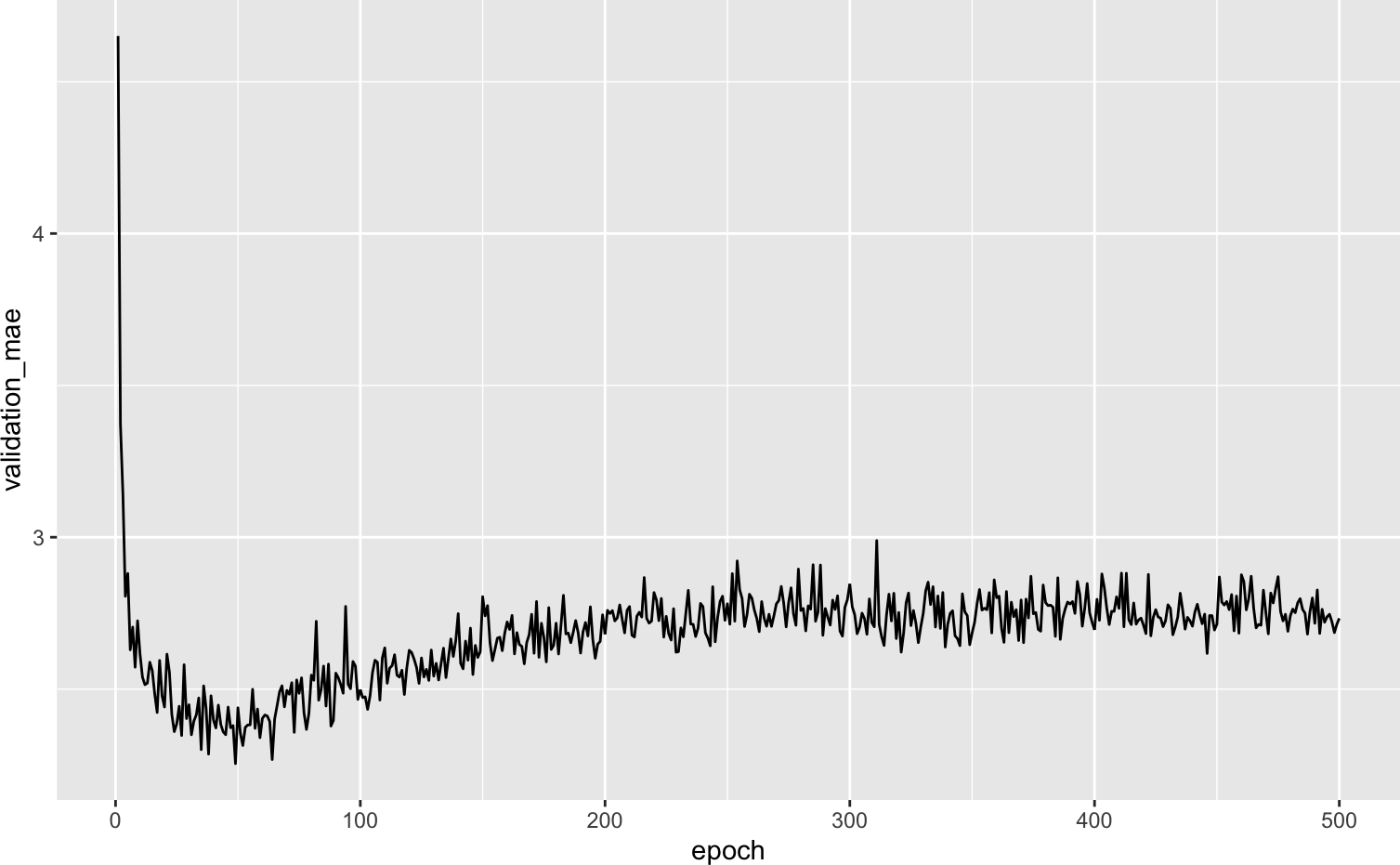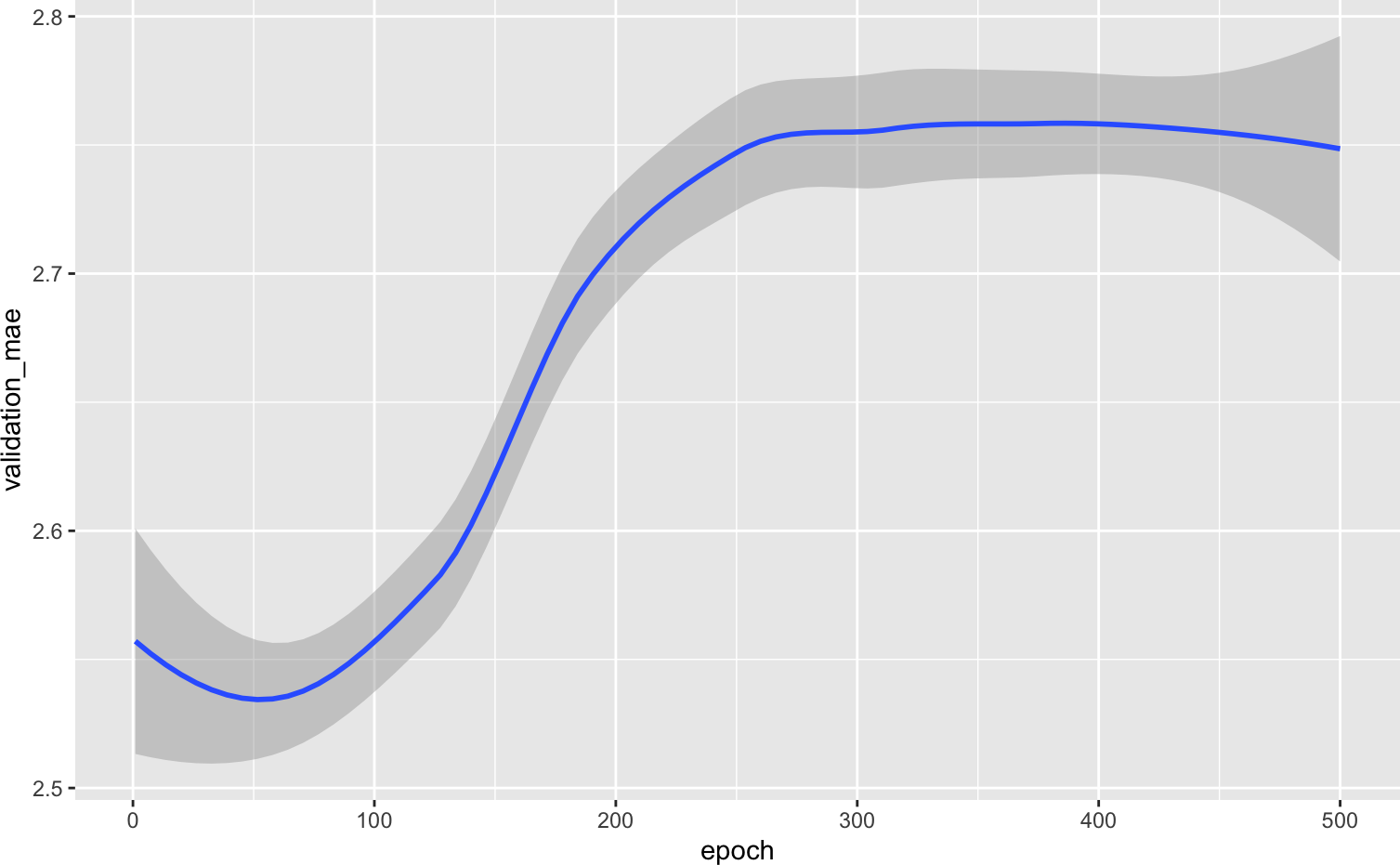# Contents

$$\newcommand{\vect}{\boldsymbol{#1}}$$ $$\newcommand{\transp}{^{\text{T}}}$$ $$\newcommand{\mat}{\boldsymbol{\mathcal{#1}}}$$ $$\newcommand{\sign}{\text{sign}}$$

This code samples are mainly from Chapter 3, Section 7 of Deep Learning with R.

# 1 The Boston Housing Price dataset

Aim: predict the median price of homes in a given Boston suburb in the mid-1970s, given a few data points about the suburb at the time, such as the crime rate, the local property tax rate, etc.

The dataset:

• 506 in total, split between 404 training samples and 102 test samples

• Each “feature” in the input data (e.g. the crime rate is a feature) has a different scale.

library(keras)

dataset <- dataset_boston_housing()
c(c(train_data, train_targets), c(test_data, test_targets)) %<-% dataset
str(train_data)
 num [1:404, 1:13] 1.2325 0.0218 4.8982 0.0396 3.6931 ...
str(test_data)
 num [1:102, 1:13] 18.0846 0.1233 0.055 1.2735 0.0715 ...

The 13 features in the input data are as follow:

1. Per capita crime rate.
2. Proportion of residential land zoned for lots over 25,000 square feet.
3. Proportion of non-retail business acres per town.
4. Charles River dummy variable (= 1 if tract bounds river; 0 otherwise).
5. Nitric oxides concentration (parts per 10 million).
6. Average number of rooms per dwelling.
7. Proportion of owner-occupied units built prior to 1940.
8. Weighted distances to five Boston employment centres.
9. Index of accessibility to radial highways.
10. Full-value property-tax rate per $10,000. 11. Pupil-teacher ratio by town. 12. 1000 * (Bk - 0.63) ** 2 where Bk is the proportion of Black people by town. 13. $$\%$$ lower status of the population. The targets are the median values of owner-occupied homes, in thousands of dollars: str(train_targets)  num [1:404(1d)] 15.2 42.3 50 21.1 17.7 18.5 11.3 15.6 15.6 14.4 ... The prices are typically between $$\ 10,000$$ and $$\ 50,000$$. If that sounds cheap, remember this was the mid-1970s, and these prices are not inflation-adjusted. ## 1.1 Preparing the data • Scale the data mean <- apply(train_data, 2, mean) std <- apply(train_data, 2, sd) train_data <- scale(train_data, center = mean, scale = std) test_data <- scale(test_data, center = mean, scale = std) ## 1.2 Building our network • very small network with two hidden layers, each with 64 units. # Because we will need to instantiate the same model multiple times, # we use a function to construct it. build_model <- function() { model <- keras_model_sequential() %>% layer_dense(units = 64, activation = "relu", input_shape = dim(train_data)[]) %>% layer_dense(units = 64, activation = "relu") %>% layer_dense(units = 1) model %>% compile( optimizer = "rmsprop", loss = "mse", metrics = c("mae") ) } For instance, a MAE of 0.5 on this problem would mean that our predictions are off by $$\500$$ on average. ## 1.3 Validating our approach using K-fold validation k <- 4 indices <- sample(1:nrow(train_data)) folds <- cut(1:length(indices), breaks = k, labels = FALSE) num_epochs <- 100 all_scores <- c() for (i in 1:4) { cat("processing fold #", i, "\n") # Prepare the validation data: data from partition # k val_indices <- which(folds == i, arr.ind = TRUE) val_data <- train_data[val_indices,] val_targets <- train_targets[val_indices] # Prepare the training data: data from all other partitions partial_train_data <- train_data[-val_indices,] partial_train_targets <- train_targets[-val_indices] # Build the Keras model (already compiled) model <- build_model() # Train the model (in silent mode, verbose=0) model %>% fit(partial_train_data, partial_train_targets, epochs = num_epochs, batch_size = 1, verbose = 0) # Evaluate the model on the validation data results <- model %>% evaluate(val_data, val_targets, verbose = 0) all_scores <- c(all_scores, results["mae"]) }  all_scores  mae mae mae mae 2.252327 2.576473 2.563274 2.586149  mean(all_scores)  2.494556 • validation scores, from 2.1 to 2.6. • average (2.37) is a much more reliable metric • We are off by $$\2,375$$ on average, which is still significant considering that the prices range from $$\10,000$$ to $$\50,000$$. • Training the network for a bit longer: 500 epochs. # Some memory clean-up k_clear_session() num_epochs <- 500 all_mae_histories <- NULL for (i in 1:k) { cat("processing fold #", i, "\n") # Prepare the validation data: data from partition # k val_indices <- which(folds == i, arr.ind = TRUE) val_data <- train_data[val_indices,] val_targets <- train_targets[val_indices] # Prepare the training data: data from all other partitions partial_train_data <- train_data[-val_indices,] partial_train_targets <- train_targets[-val_indices] # Build the Keras model (already compiled) model <- build_model() # Train the model (in silent mode, verbose=0) history <- model %>% fit( partial_train_data, partial_train_targets, validation_data = list(val_data, val_targets), epochs = num_epochs, batch_size = 1, verbose = 0 ) mae_history <- history$metrics\$val_mae
all_mae_histories <- rbind(all_mae_histories, mae_history)
}

We can then compute the average of the per-epoch MAE scores for all folds:

average_mae_history <- data.frame(
epoch = seq(1:ncol(all_mae_histories)),
validation_mae = apply(all_mae_histories, 2, mean)
)

Let’s plot this:

library(ggplot2)
ggplot(average_mae_history, aes(x = epoch, y = validation_mae)) + geom_line()It may be a bit hard to see the plot due to scaling issues and relatively high variance. Let’s use geom_smooth() to try to get a clearer picture:

ggplot(average_mae_history, aes(x = epoch, y = validation_mae)) + geom_smooth()• It seems that validation MAE stops improving significantly after 70 epochs.
• Past that point, we start overfitting.

## 1.4 Final model at epoch 80

# Get a fresh, compiled model.
model <- build_model()

# Train it on the entirety of the data.
model %>% fit(train_data, train_targets,
epochs = 80, batch_size = 16, verbose = 0)

result <- model %>% evaluate(test_data, test_targets)
result
     loss       mae
19.405186  2.737695 

We are still off by about $$\2,680$$.